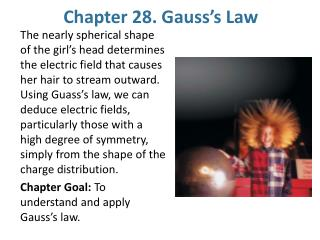# Chapter 28. Gauss’s Law - PowerPoint PPT PresentationDownload PresentationChapter 28. Gauss’s Law

Chapter 28. Gauss’s LawDownload Presentation## Chapter 28. Gauss’s Law

- - - - - - - - - - - - - - - - - - - - - - - - - - - E N D - - - - - - - - - - - - - - - - - - - - - - - - - - -
##### Presentation Transcript

1. Chapter 28. Gauss’s Law The nearly spherical shape of the girl’s head determines the electric field that causes her hair to stream outward. Using Guass’s law, we can deduce electric fields, particularly those with a high degree of symmetry, simply from the shape of the charge distribution. Chapter Goal: To understand and apply Gauss’s law.

2. Stop to think 28.2 page 855 Stop to think 28.3 page 861 Stop to think 28.4 page 865 Stop to think 28.5 page 869

3. Electric Field

4. Symmetry

5. Gaussian Surface

6. The Electric Flux through a Closed Surface The electric flux through a closed surface is The electric flux is still the summation of the fluxes through a vast number of tiny pieces, pieces that now cover a closed surface. NOTE: A closed surface has a distinct inside and outside. The area vector dA is defined to always point toward the outside. This removes an ambiguity that was present for a single surface, where dA could point to either side.

7. These are two-dimensional cross sections through three-dimensinal closed spheres and a cube. Rank in order, from largest to smallest, the electric fluxes Фa to Фe through surfaces a to e. Фb = Фe > Фa = Фc = Фd

8. Conductors in Electrostatic Equilibrium The electric field is zero at all points within a conductor in electrostatic equilibrium. If this weren’t true, the electric field would cause the charge carriers to move and thus violate the assumption that all the charges are at rest. The electric field at the surface of a charge carrier is where η is the surface charge density of the conductor.

9. Which Gaussian surface would allow you to use Gauss’s law to determine the electric field outside a uniformly charged cube? • A cube whose center coincides with the  center of the charged cube and which has  parallel faces. • A sphere whose center coincides with the  center of the charged cube. • Neither A nor B. • Either A or B.

10. Example 28.4 Inside a sphere of change ρ is charge volume density = Q/(4/3)πa3